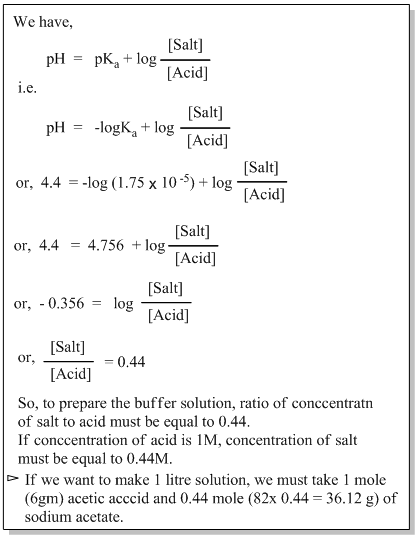• Post Author:

#### What is buffer solution?

A solution which can resist the change in pH even on addition of small amount of acid or base is called buffer solution.

Example: In a biological system, blood is an example of buffer and its pH remains almost constant to 7.4 (i.e. 7.35-7.45). Blood contains a buffer of carbonic acid (H2CO3) and bicarbonate anion (HCO3).

#### Types of buffer solution

1. Acidic buffer solution: The buffer solution prepared by mixing equimolar quantities of weak acid and its salt with strong base is known as acidic buffer solution.

The pH value of acidic buffer solution is less than 7.

Example: A solution containing equimolar quantities of acetic acid (CH3COOH) and sodium acetate (CH3COONa) is acidic buffer.

2. Basic buffer solution: The buffer solution prepared by mixing equimolar quantities of weak base and its salt with strong acid is known as basic buffer solution.

The pH value of acidic buffer solution is more than 7.

Example: A solution containing equimolar quantities of ammonium hydroxide (NH4OH) and ammonium chloride (NH4Cl) is basic buffer.

#### Mechanism of buffer action

Mechanism of buffer solution can be explained by considering acidic buffer solution as:

• Suppose, an acidic buffer prepared by adding equimolar quantities of CH3COOH and CH3COONa, where CH3COOH is weakly ionized and CH3COONa is strongly ionized.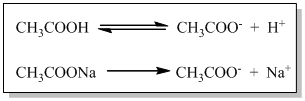• If we add few drops of acid (HCl) to the buffer solution then it provides H+ ions to the buffer. These H+ ions would combine with CH3COO ions present in the buffer solution to form weakly ionized acetic acid as,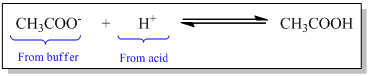• Similarly, if we add small amount of NaOH to the buffer solution then it provides OH ions to the buffer. These OH ions would combine with H+ ions present in the buffer solution to form undissociated H2O molecules.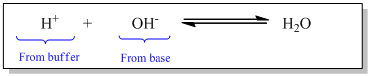• Thus, addition of H+ ions is neutralized by CH3COO ions and OH is neutralized by H+ ions. Therefore, pH of the solution remains unchanged.

#### Buffer capacity and Buffer range

Buffer capacity : Buffer capacity can be defined as the ability of a solution to resist rapid changes in pH.

In other words, buffer capacity is defined as the number of moles of acid or base added per litre of the buffer required to cause a unit change in pH.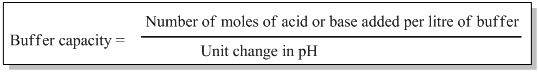Buffer range: A buffer solution can be designed for any pH range, but a given buffer solution will work effectively only over a particular range.

The pH range over which a buffer solution is effective is termed as buffer range. For acidic buffer solution approximate pH range is pH=pKa±1 and pOH=pKb±1 for basic buffer solution.

#### Applications of buffer solution

Buffer solutions are important in various fields of science and industry due to their ability to maintain a stable pH level. Some of their key applications are:

1. Laboratory work: Buffers are essential in chemical and biological laboratories to maintain constant pH during experiments and reactions. This helps to ensure the accuracy and reproducibility of results.
2. Analytical chemistry: Instruments like ph meter depend on buffer solutions to calibrate and accurately measure the pH of other solutions.
3. Medicinal and clinical diagnostics: Buffers are used in various medical diagnoses, including blood tests.
4. Pharmaceuticals: Buffers are used in the formulation of drugs to ensure the stability and effectiveness of drugs, especially in intravenous medicines.
5. Water treatment: Buffer solutions are sometimes used to adjust and maintain the pH of water in swimming pools, aquariums and industrial water treatment processes.

##### Handerson-Hasselbalch equation – Determination of pH of buffer

Handerson-Hasselbalch equation is a formula used to calculate the pH of a buffer solution. It is expressed as: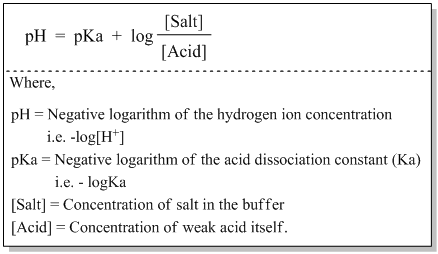Derivation of Handerson-Hasselbalch equation :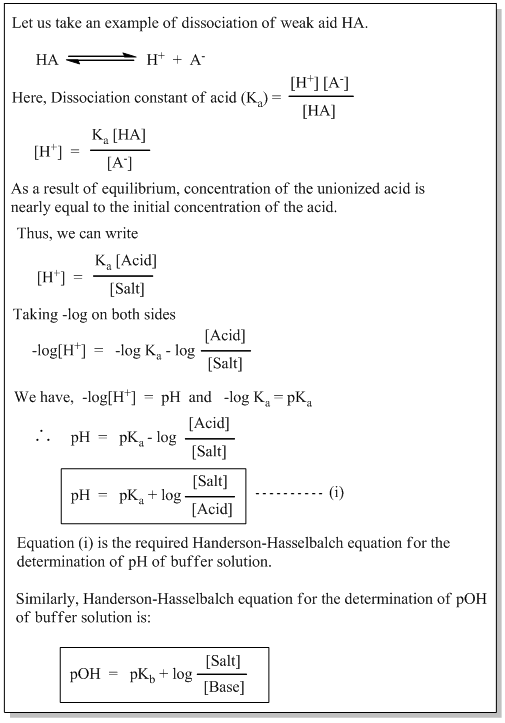#### Preparation of buffer solution

Handerson-Hasselbalch equation can be used for the calculation while preparing buffer solution. Example:

Q) The dissociation constant of acetic acid is 1.75×10-5. How will you prepare a buffer solution of 4.4 by mixing sodium acetate and acetic acid.

Ans..